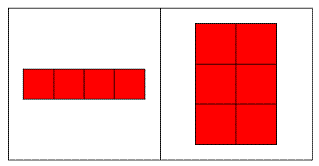# Cell Arrays : variables of different size in a programming environment

Cell arrays are able to store variables of different size or type (very much like structures in other programming languages). It's the most versatile data object.

This type of array in Matlab uses integer indices, just like an ordinary n-dimensional array of only numbers. An array like this, use braces as indexing operators ({}).

As an example, the following line creates the so called cell array (CA) containing the string ‘any string’ as element one (that is a{1}), and a 3-by-4 random matrix as element two (that is a{2}):

a = {'any string', rand(3, 4)};

A CA can be indexed, so the variable created in the previous example can be indexed like this:

a{1}
ans = any string

a{2}
ans =     0.7382    0.9355    0.8936
0.1763    0.9169    0.0579
0.4057    0.4103    0.3529

The indexing operator can also be used to insert or overwrite elements of a CA. The following code inserts the scalar number 5.3 on the third place of the previously created array:

a{3} = 5.3;

### Creating Cell Arrays

The example above showed how to create an array containing currently available or created variables. In many situations it is useful to create a CA and then fill it with data.

The cell function returns an array of a given size, containing empty matrices. The following line creates a 3-by-2 array containing empty matrices:

a = cell(3,2)

produces

a =
[]     []
[]     []
[]     []

Just like numerical matrices, CA can be multidimensional. The cell function accepts a number of positive integers to describe the size of the returned array. In the following example an array is created, and its size is displayed:

a = cell(3, 4, 5);
size(a)

(produces ans = 3     4     5)

As an alternative to creating empty cell arrays, and then filling them, it is possible to convert numerical arrays into cell arrays using the num2cell and mat2cell functions (type ‘help num2cell’ or ‘help mat2cell’ on the command screen to view an explanation of these built-in functions).

### Indexing Cell Arrays

Elements can be inserted into CA using braces ({}). Indexing works for these arrays like for matrices.

Accessing values in a CA is different from the same operation for numerical arrays. For example, let’s define these three matrices:

m1 = [1 2 3]
m2 = [9 8 7 6]
m3 = [5 6; 6 1; 9 0]

We can create a cell array like this:

c = {m1 m2 m3}

this produces

c = [1x3 double]    [1x4 double]    [3x2 double]

and we can access specific values in this way:

c{1}
(produces ans = 1     2     3)

c{3}

produces

ans =
5     6
6     1
9     0

c{3}(3,1)
(produces ans = 9)

### Cell Arrays of Strings

A common use of CA is to store multiple strings in the same variable. It is possible to store multiple strings in a matrix by letting each row be a string. This, however, introduces the problem that all strings must be of equal length. Therefore it is recommended to use cell arrays to store multiple strings. If, however, the character matrix representation is required for an operation, it can be converted to a cell array of strings using the cellstr function.

These five lines of code

m = ['hello'; 'world'];
c = cellstr(m)
size(c)
c(1)

c(2)

produce these results:

c = 'hello'
'world'
ans = 2     1
ans = 'hello'
ans = 'world'

### Manipulating Cells

There are several functions available for cell manipulation. Some of these are cellstr, iscellstr, cell2struct, struct2cell, iscell, num2cell, etc. Type help function on the command screen to see more details regarding the use of those functions.

cellplot plots the cell array schematically, so that we can understand how and where we are saving our data...

This 4-line code

m2 = [9 8 7 6];
m3 = [5 6; 6 1; 9 0];
c = {m2 m3};

cellplot(c)

produces this image:so that we can better visualize how we are handling our data.

From 'Cell arrays' to home

From 'Cell arrays' to 'Matlab Tutorials'